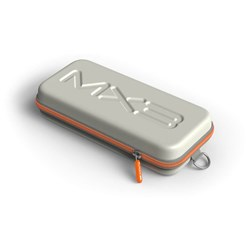# Hydration / Nutrition

Grid List
\$92.52
Increase value Decrease value
\$147.29
Increase value Decrease value
\$19.97
Increase value Decrease value
\$26.31
Increase value Decrease value
\$26.31
Increase value Decrease value
\$26.31
Increase value Decrease value
Atago Refractometer Master SUR/NM 2773
\$735.60
Increase value Decrease value
\$47.98
Increase value Decrease value
\$29.02
Increase value Decrease value
\$29.02
Increase value Decrease value
\$29.02
Increase value Decrease value
\$1499.00
Increase value Decrease value
\$45.76
Increase value Decrease value\$33.00
Increase value Decrease value
\$29.02
Increase value Decrease value
\$874.50
Increase value Decrease value
\$36.25
Increase value Decrease value
\$47.98
Increase value Decrease value
\$36.25
Increase value Decrease value
\$189.55
Increase value Decrease value
\$28.95
Increase value Decrease value
\$49.95
Increase value Decrease value
From
Increase value Decrease value
From
Increase value Decrease value
Grid List
##### Products to compare:
Comparing Products